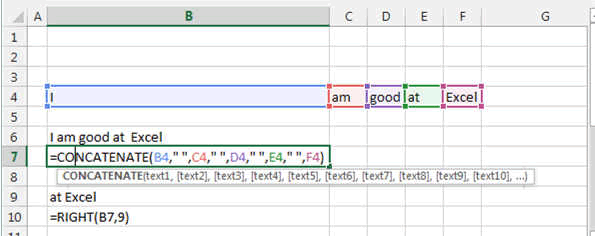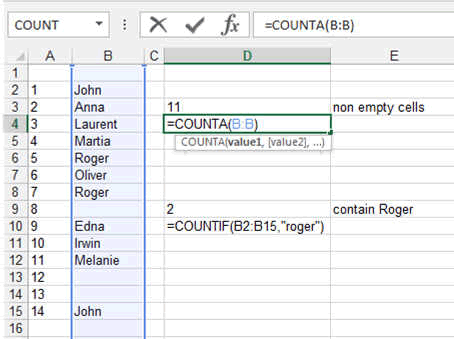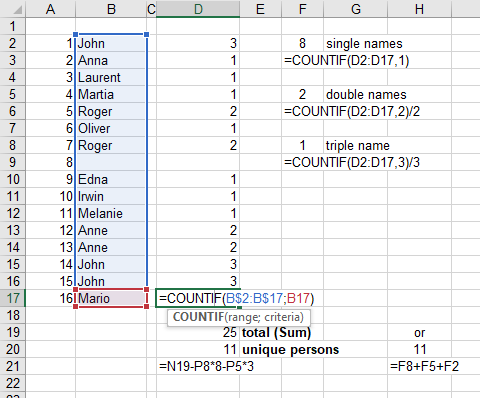## Play with Words in Excel

In Excel you can play with Words. That is quite funny actually.

In Excel you will be entering numbers and texts in your spreadsheet, then you will want to do something with all this data you entered. For example you would want to extract part of the sentences that are in a cell or you might want to add two cells together. All this is possible with Excel text and string functions.

We will give you here four examples of the most used Excel string functions. You can go in our section for advanced text handling (see menu advance chapter Excel Advanced Text Handling) or in the top menu function and there you will find all the texts functions and an example for each of the Excel Functions.

You can add words, cut words, extract words.:

The main function are called:

CONCATENATE: which puts words together. Here you type CONCATENATE(cell1, cell2, cell3, "another word", cell4, ....)

LEFT: takes n characters from the Left of the word or sentence. LEFT(cell including the string, a number)

RIGHT: takes n characters from the Right of the word or sentence. RIGHT(cell including the string, a number)

MID: returns a specific number of characters from a word, sentence or text string, starting at the position you specify, based on the number of characters you specify. MID(cell including the string, number representing the first letter you want, and then the lastone).

Here an Exemple:### Number of words in an Excel Cell

To count the number of words in a Cell, Excel did not develop a single formula. Nevertheless, you can use the combination of TRIM, LEN and SUBSTITUTE to do this.

Enter the count word formula in a cell

=IF(LEN(TRIM(A1))=0,0,LEN(TRIM(A1))-LEN(SUBSTITUTE(A1," ",""))+1)

This is quite useful if you decide to write a lot of text in Excel.

### Number of word, Counting word or line or non/empty cells

This is a so important function that I had to put it here in the basics although it is not so basic.

Sometimes you have a long list of items or name and you want to know how many are non empty or repeat themselves.

Here how to:

Take this list of names. We want to know how many are in total inside and how many ROGER there are.

The function COUNTIF is used here. This COUNTIF function allows you to enter a condition to the counting.

Here we look for the word "roger". So it become COUNTIF(B2:B15, "roger").

You can enter more complex conditions like COUNTIF(B2:B15, ">3") : this means it will look at how many cells in the range B2:B15 are bigger than 3.

COUNTIF(B2:B15, "<Today()") : you can guess here, it will count entries that are older than the ones with TODAY's date

The Excel Function COUNTA function counts the number of NON-EMPTY cells in an area. COUNTA(B2:C15) counts the non empty cells in the B2 to C15 range.REMARK:

Look at the formulas. The first one shows the range B:B which means the full B column.

The second one show a specific range B2:B15.

You can use both ways here but not always. If you have a header, using B:B will include the header so you should use B2:Bxxx

Let's do some magic Excel Trick.

Lets count how many unique people are in a list

Here we use the function COUNTIF many time.

The first time in the column D where next to each name we use the COUNTIF to search this name in the column with COUNTIF(B\$2:B\$17, the cell next to the name).

Then we see that no name is here more than 3 time. So we use COUNTIF again in column F to count the number 1, 2, 3. And finally we sum up these three numbers to have the total number of unique person on the list.

We could use a pivot table too to count the number of occurence of a word or number.These were some basics. Now you will learn by doing.